# Hit-or-miss topology

Jump to: navigation, search

This scheme for introducing a topology into a collection of sets (cf. [a1], [a5], [a9]) can be described conveniently by the "hit or miss" metaphor. Given a topological spaceand a collectionof sets in, one introduces a topological structure (topology)ondepending on familiesof sets in, where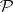is closed under finite unions, by taking as an open base for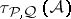the family of sets of the form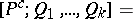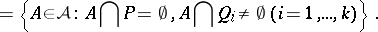The basic open setcollects those sets inthat "miss"and "hit" every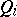.

Important realizations of this scheme are:

1) the exponential topology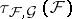(cf. [a1], [a5], [a9]);

2) the hit-or-miss topology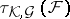(cf. [a4]);

3) the myope topology(cf. [a4]). Here,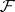is the collection of all closed sets in,is the collection of all open sets in, andis the collection of all compact sets in.

The hit-or-miss topology is an important tool in mathematical morphology (cf. [a4], [a7]) in Euclidean spaces, hence one most often considers locally compact metric spaces(cf. Locally compact space; Metric space). The topological space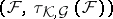is a compact metric space (cf. [a4]); the topology of this space can be described (cf. [a4]) in terms of convergent sequences: A sequenceconverges into a setif and only if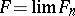(meaning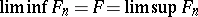, where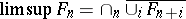and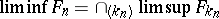; see [a3]).

The relations of the hit-or-miss topology 2) to the exponential topology 1) and myope topology 3) can be briefly summarized as follows. In general, the exponential topology is finer than the hit-or-miss topology 2) and the myope topology is finer than the restrictionof the hit-or-miss topology to the collection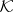; the myope topology and the topologycoincide on any subspacethat is compact in the myope topology (compactness of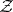means thatis closed in the hit-or-miss topology and there exists a compact set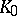such thatfor any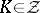; cf. [a4]).

The Hausdorff metric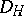on the collectionis given by (cf. [a2], [a3]):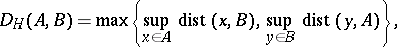where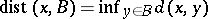(a bounded metric on).

The topology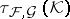is metrizable (cf. Metrizable space) byrestricted to(cf. [a3]); hence the hit-or-miss topology on any subspacethat is compact in the myope topology is metrizable by the metric.

A mapping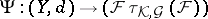from a metric spaceintois upper semi-continuous (cf. also Semi-continuous mapping) if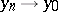impliesAn illustration is provided by the basic mappings of mathematical morphology in a Euclidean space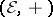(cf. [a4], [a7]): the opening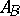and the closing(cf. also Mathematical morphology). Both mappings are upper semi-continuous in the hit-or-miss topology (cf. [a4]). The property of upper semi-continuity implies stability of either of these mappings in the morphological sense (cf. [a7]).

A ramification of the hit-or-miss topology was introduced into collections of rough sets generated from information systems (cf. [a6]) to yield a counterpart of mathematical morphology on abstract data sets (see also [a8]).